16.4 C
New York
Thursday, June 8, 2023
HomeData ScienceTop 10 Deep Learning Algorithms That Every AI Enthusiast Should Know

# Top 10 Deep Learning Algorithms That Every AI Enthusiast Should Know

Deep Learning is basically a subset of Artificial Intelligence and Machine Learning. Typical AI and ML algorithms can work with datasets having a few hundred features. However, an image or a signal may have millions of attributes. That’s where the Deep Learning Algorithms come in. Most of the DL algorithms have been inspired by the human brain called the artificial neural network. The modern world has extensive use of Deep Learning. From biomedical engineering to simple image processing – it has its uses. If you want to become an expert in this field, you have to go through the different DL algorithms. And that’s what we will be discussing today.

## Top Deep Learning Algorithms

The use of Deep Learning has highly increased in most fields. Deep learning is reasonably practical when working with unstructured data because of its capacity to process vast amounts of features. Different algorithms are suitable for solving different problems. To make yourself acquainted with the different DL algorithms, we will list the top 10 Deep Learning algorithms you should know as an AI enthusiast.

### 01. Convolutional Neural Network (CNN)

CNN is perhaps the most popular neural network for image processing. A CNN generally takes an image as input. The neural network analyzes each pixel separately. The weights and biases of the model are then tweaked to detect the desired object from the image. Like other algorithms, the data also has to pass through pre-processing stage. However, a CNN needs relatively less pre-processing than most other DL algorithms.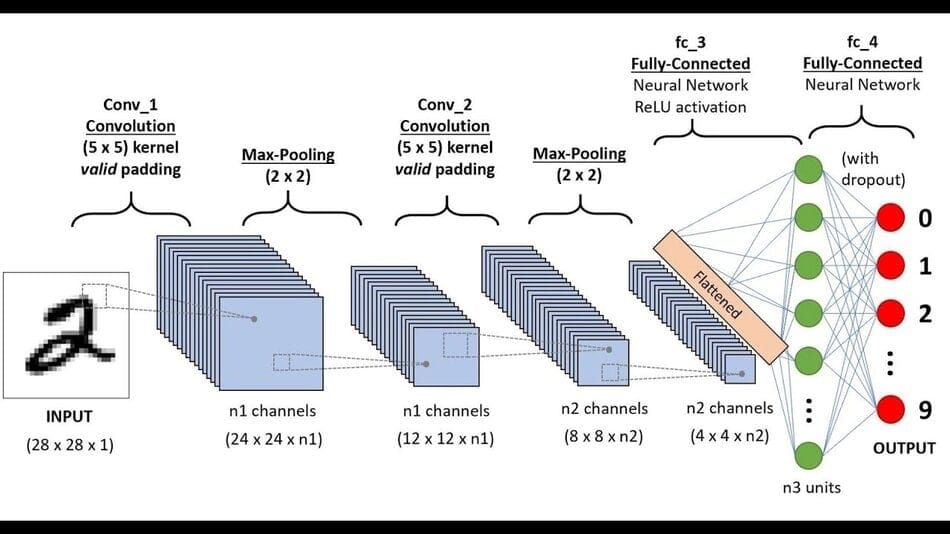Key Features

• In any computer vision algorithm, the image or signal has to go through a filtering process. CNN has many convolutional layers to do this filtering.
• After the convolutional layer, there remains a ReLU layer. It stands for Rectified Linear Unit. It conducts operations on the data and outputs a rectified attribute map.
• We can find a rectified feature map from the ReLU layer. It then goes through the pooling layer. So it is basically a sampling method.
• The pooling layer reduces the dimension of the data. Reducing dimensions makes the learning process comparatively less expensive.
• The pooling layer flattens two-dimensional matrices from the aggregated feature vector to create a single, lengthy, prolonged, sequential vector.
• The fully connected layer comes after the pooling layer. The fully connected layer basically has some hidden neural network layers. This layer classifies the image into different categories.

### 02. Recurrent Neural Networks (RNNs)

RNNs are a sort of neural network in which the outcome from the previous phase is passed into the present phase as input. For classic neural networks, the input and output are not interdependent. However, when you need to predict any word in a sentence, the previous word needs to be considered. Prediction of the next word is not possible without remembering the last word. RNNs came into the industry to solve these types of problems.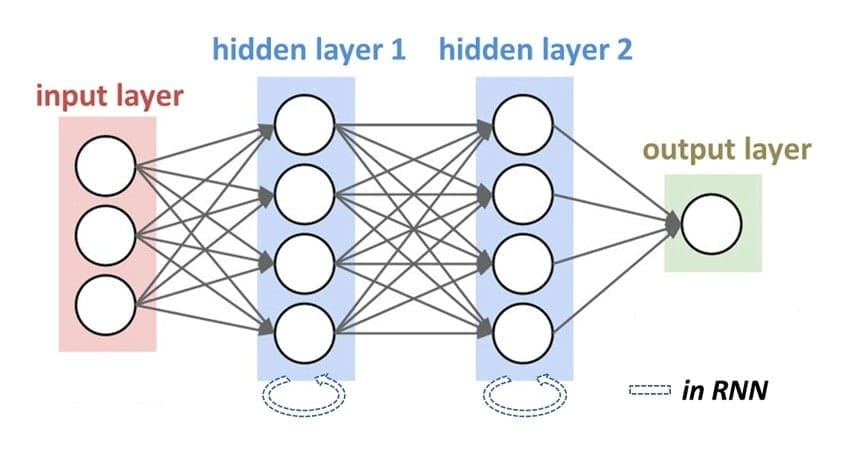Key Features

• The hidden state, which stores certain details about a cycle, is the essential element of RNN. Nevertheless, RNN’s basic characteristics depend on this state.
• RNNs possess a “memory” that stores all data about the calculations. It employs the same settings for each entry since it produces the same outcome by performing the same command on all intakes or hidden layers.
• RNN reduces the complication by converting autonomous activations into dependent ones by giving all levels the same biases and weights.
• As a result, it simplifies the learning process by upgrading the parameters and remembering previous results by feeding each outcome into the next hidden level.
• Furthermore, all these layers can be combined into a single recurrent layer, with the biases and weights of all the hidden layers being the same.

### 03. Long Short Term Memory Networks (LSTMs)

Recurrent Neural Networks or RNNs basically work with voice-related data. However, they don’t work well with short-term memory. They’ll have difficulty transporting info from one step to other steps if the chain is lengthy sufficiently. If you’re trying to forecast something from a passage of content, RNNs might miss out on critical information. To solve this issue, researchers developed a modern version of RNN called LSTM. This Deep Learning algorithm rules out the short-term memory issue.

Key Features

• LSTMs keep track of data throughout time. Since they can trace past data, they are valuable in solving time-series problems.
• Four active layers integrate in a special manner in LSTMs. As a result, the neural networks possess a structure like a chain. This structure allows the algorithm to extract small information from the content.
• The cell state and its many gates are at the heart of LSTMs. The cell state serves as a transportation route for relevant data as it travels down the sequential chain.
• Theoretically, the cell state can retain necessary details all through the sequence’s execution. As a result, data from previous steps can find its way to subsequent time steps, lessening the short-term memory impacts.
• Besides time series prediction, you can also use LSTM in the music industry, speech recognition, pharmaceutical research, etc.

### 04. Multilayer Perceptron

A point of entry into complicated neural networks, where input data routes through multiple levels of artificial neurons. Each node is linked to every other neuron in the upcoming layer, resulting in an entirely joined neural network. The input and output layers are available, and a hidden layer is present between them. That means each multilayer perceptron has at least three layers. Furthermore, it has multimodal transmission, which means it can propagate both forward and backward.

Key Features

• Data goes through the input layer. Then, the algorithm multiplies the input data with their respective weights in the hidden layer, and the bias is added.
• The multiplied data then passes to the activation function. Different activation functions are used according to the input criteria. For example, most data scientists use the sigmoid function.
• Moreover, there is a loss function to measure the error. The most commonly used are log loss, mean squared error, accuracy score, etc.
• In addition, the Deep Learning algorithm uses the backpropagation technique to reduce the loss. The weights and biases are then changed through this technique.
• The technique continues until the loss becomes a minimum. At the minimum loss, the learning process is said to be finished.
• Multilayer perceptron has many uses, such as complex classification, speech recognition, machine translation, etc.

### 05. Feed Forward Neural Networks

The most basic type of neural network, in which input information goes in one direction only, entering through artificial neural nodes and leaving via output nodes. In areas where hidden units may or may not be present, incoming and outgoing layers are available. Relying on this, one can classify them as a multilayered or single-layered feedforward neural network. Since FFNNs has a simple architecture, their simplicity can be advantageous in certain machine learning applications.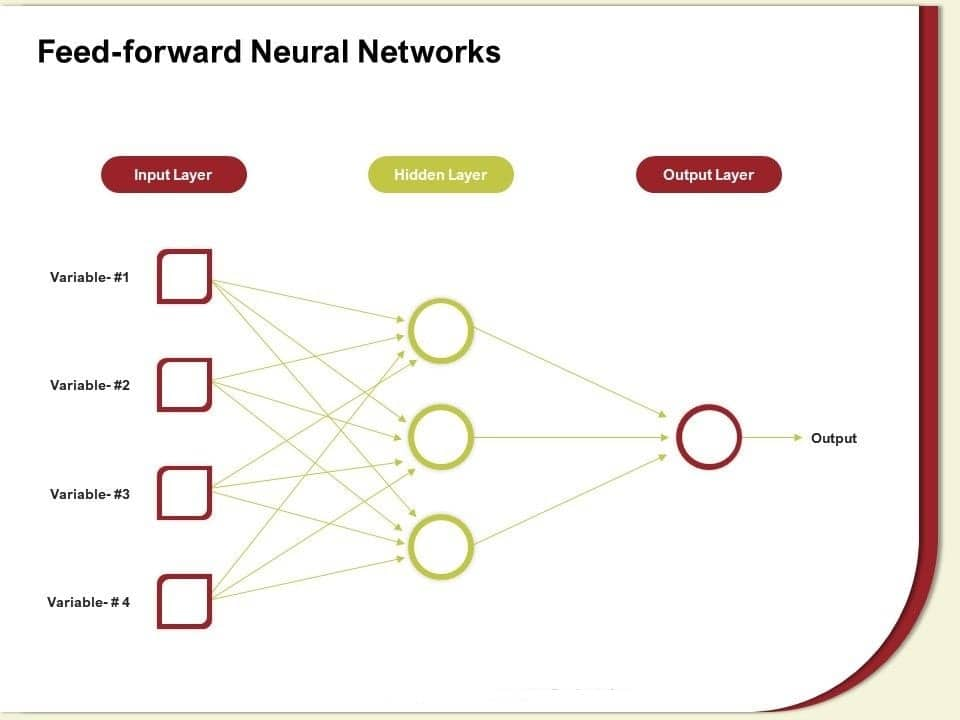Key Features

• The function’s sophistication determines the number of layers. Upward transmission is unidirectional, but there is no backward propagation.
• Moreover, the weights are fixed. Inputs are combined with weights and sent into an activation function. A classification or step activation function is utilized to do this.
• If the addition of the readings is more than a predetermined threshold, which is normally set at zero, the outcome is generally 1. If the sum is less than the threshold, the output value is generally -1.
• The Deep Learning algorithm may evaluate the outcomes of its nodes with the desired data using a technique known as the delta rule, enabling the system to alter its weights during learning to create more precise output values.
• However, the algorithm doesn’t have any dense layers and backward propagation, which is not suitable for computationally expensive problems.

### 06. Radial Basis Function Neural Networks

A radial basis function analyzes any point’s span from the center. There are two levels to these neural networks. First, the attributes merge with the radial basis function in the inner layer. Then, when computing the same outcome in the next layer, the output of these attributes is considered. In addition to that, the output layer has one neuron for each category. The algorithm uses the similarity of the input to sample points from the training data, where each neuron maintains a prototype.

Key Features

• Each neuron measures the Euclidean distance between the prototype and the input when a fresh input vector, i.e., the n-dimensional vector you’re attempting to categorize, needs to be classed.
• After the comparison of the input vector to the prototype, the algorithm provides an output. The output usually ranges from 0 to1.
• The output of that RBF neuron will be 1 when the input matches the prototype, and as the space between the prototype and input increase, the results will move towards zero.
• The curve created by neuron activation resembles a standard bell curve. A group of neurons makes up the output layer.
• In power restoration systems, engineers often utilize the radial basis function neural network. In an attempt to reestablish power in the lowest amount of time, people use this neural network in power restoration systems.

### 07. Modular Neural Networks

Modular Neural Networks combine several Neural Networks to resolve an issue. In this case, the different neural networks act as modules, each solving a portion of the problem. An integrator is responsible for dividing the issue into numerous modules as well as integrating the answers of the modules to form the program’s ultimate output.

A simple ANN cannot provide adequate performance in many cases in response to the problem and needs. As a result, we may require multiple ANNs to address the same challenge. Modular Neural Networks are really great in doing this.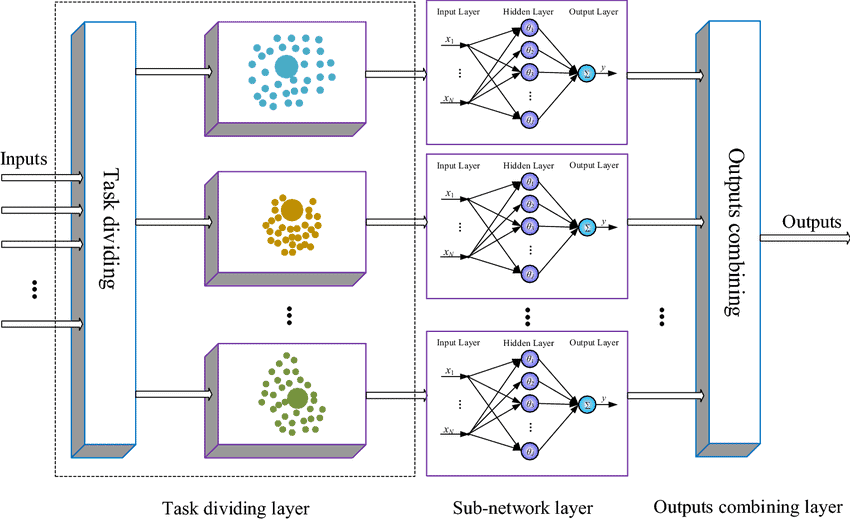Key Features

• Various ANNs are used as modules in MNN to address the entire problem. Each ANN symbolizes a module and is in charge of tackling a certain aspect of the problem.
• This method entails a collaborative effort among the numerous ANNs. The goal at hand is to divide the problem into different modules.
• Each ANN or module is provided with certain input according to its function. The numerous modules each handle their own element of the problem. These are the programs that compute the findings.
• An integrator receives the analyzed results. The integrator’s job is to integrate the numerous individual replies from the numerous ANNs and produce a combined answer that serves as the system’s output.
• Hence, the Deep Learning algorithm solves the issues by a two-part method. Unfortunately, despite numerous uses, it is not suitable for moving target problems.

### 08. Sequence-To-Sequence Models

Two Recurrent Neural Networks make up a sequence to sequence model. There is an encoder for processing the data and a decoder for processing the result here. The encoder and decoder both work at the same time, utilizing the same or separate parameters.

In contrast to the real RNN, this model is especially useful when the quantity of the input data and the size of the output data are equal. These models are primarily used in question answering systems, machine translations, and chatbots. However, the advantages and disadvantages are similar to that of RNN.

Key Features

• Encoder-Decoder architecture is the most basic method to produce the model. This is because both the encoder and decoder are actually LSTM models.
• The input data goes to the encoder, and it transforms the whole data input into internal state vectors.
• This context vector seeks to encompass the data for all input items to aid the decoder in making correct forecasts.
• In addition, the decoder is an LSTM whose starting values are always at Encoder LSTM’s terminal values, i.e., the context vector of the encoder’s last cell goes into the decoder’s first cell.
• The decoder generates the output vector using these beginning states, and it takes these outcomes into account for subsequent responses.

### 09. Restricted Boltzmann Machines (RBMs)

Geoffrey Hinton developed Restricted Boltzmann Machines for the first time. RBMs are stochastic neural networks that can learn from a probabilistic distribution over a collection of data. This Deep Learning algorithm has many uses like feature learning, collaborative filtering dimensionality reduction, classification, topic modeling, and regression.

RBMs produce the basic structure of Deep Belief Networks. Like many other algorithms, they have two layers: the visible unit and the hidden unit. Each visible unit joins with all the hidden units.Key Features

• The algorithm basically works with the combination of two phases. These are the forward pass and the backward pass.
• In the forward pass, RBMs receive the data and convert those into a set of numbers that encodes the inputs.
• RBMs integrate each input with its own weighting and a single overall bias. Finally, the output is passed to the hidden layer by the technique.
• RBMs acquire that collection of integers and transform them to generate the recreated inputs in the backward pass.
• They mix each activation with its own weight and overall bias before passing the result to the visible layer for rebuilding.
• The RBM analyzes the reconstructed data to the actual input at the visible layer to assess the effectiveness of the output.

### 10. Autoencoders

Autoencoders are indeed a sort of feedforward neural network where the input and output are both similar. In the 1980s, Geoffrey Hinton created autoencoders to handle unsupervised learning difficulties. They’re neural networks that repeat inputs from the input layer to the output layer. Autoencoders have a variety of applications, including drug discovery, image processing, and popularity prediction.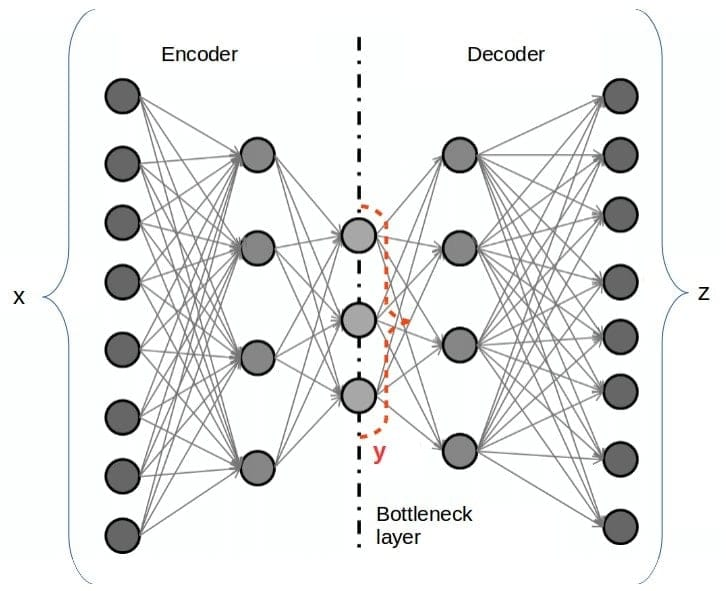Key Features

• Three layers comprise the Autoencoder. They are the encoder coder, the code, and the decoder.
• Autoencoder’s design allows it to take in information and turn it into a different context. Then they try to recreate the real input as accurately as possible.
• Sometimes, data scientists use it as a filtering or segmentation model. For example, suppose an image is not clear. Then, you can use an Autoencoder to output a clear image.
• Autoencoders encode the picture first, then compress the data into a smaller form.
• Finally, the Autoencoder decodes the image, which produces the recreated image.
• There are various types of encoders, each of which has its respective use.

## Ending Thoughts

Over the last five years, Deep Learning algorithms have grown in popularity across a wide range of businesses. Different neural networks are available, and they work in separate ways to produce separate results.

With additional data and use, they will learn and develop even more. All these attributes have made deep learning famous among data scientists. If you want to dive into the world of computer vision and image processing, you need to have a good idea of these algorithms.

So, if you want to enter into the fascinating field of data science and gain more knowledge of Deep Learning algorithms, have a kick start and go through the article. The article gives an idea about the most famous algorithms in this field. Of course, we couldn’t list all the algorithms but only the important ones. If you think we have missed anything, let us know by commenting below.Mehedi Hasan
Mehedi Hasan is a passionate enthusiast for technology. He admires all things tech and loves to help others understand the fundamentals of Linux, servers, networking, and computer security in an understandable way without overwhelming beginners. His articles are carefully crafted with this goal in mind - making complex topics more accessible.